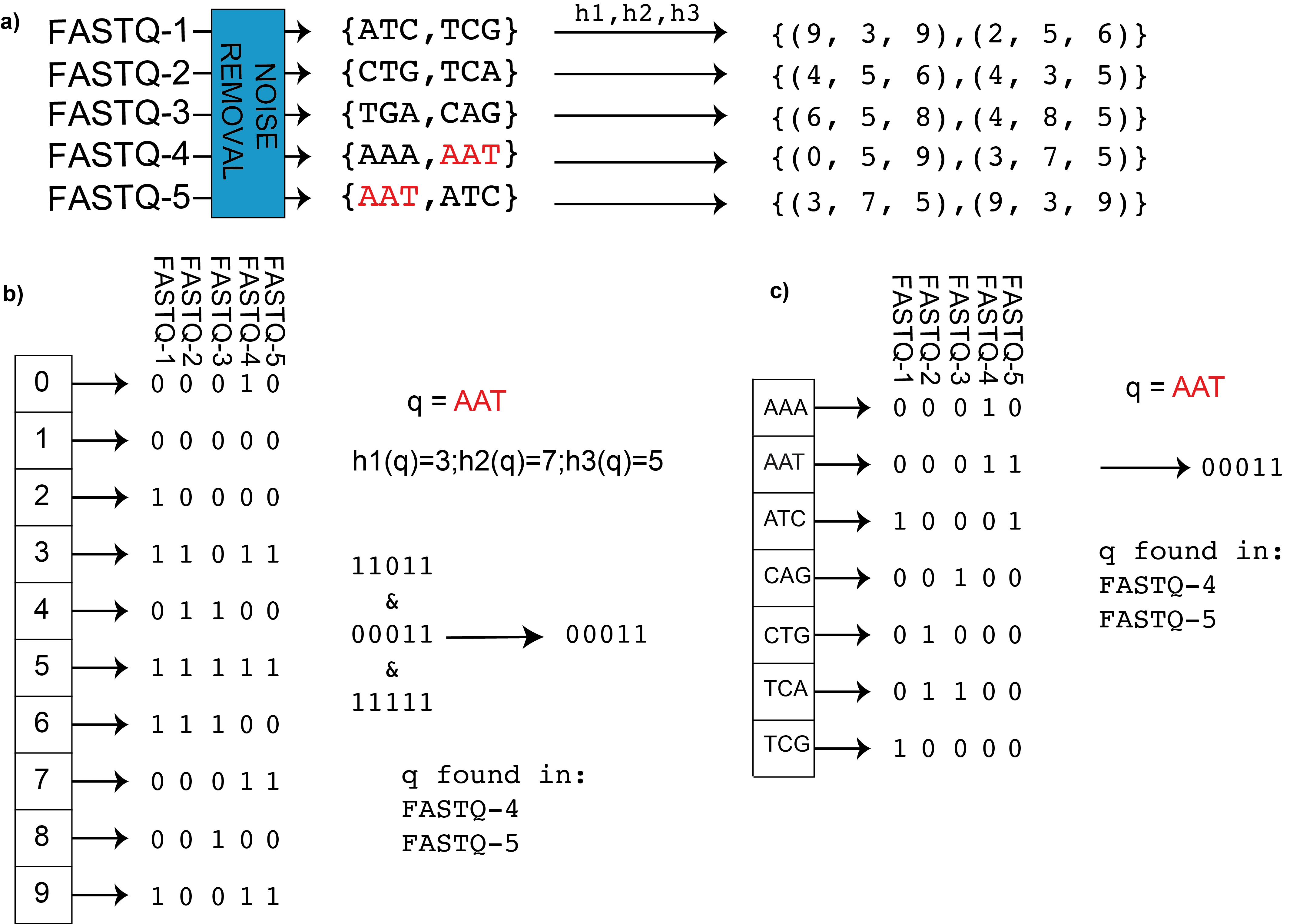### Tutorials

View All 6## What is BIGSI?

BIGSI indexes a set of N bloom filters by position in the bloom filter. Each bloom filter must be constructed with the same parameters (m, η), where m is the bloom filter’s length in bits and η is the number of hash functions applied to each k-mer. The same hash functions must also be used to construct each bloom filter. To construct a BIGSI, the N bloom filters are column-wise concatenate into a matrix. The row index and row bit-vectors are then inserted into a hash table or key-value store as key-value pairs so that row lookups can be done in O(1) time. This set of key-value pairs can be stored on disk, in memory or distributed across several machines. To insert a new bloom filter we simply append it as a column to the existing bitmatrix.
To query the BIGSI for a k-mer we hash the k-mer η times, look up the resulting keys in the key-value store, and take the bit-wise AND of the resulting bit-vectors.

In the figure we can see BIGSI encoding compared with naïve approach. a) BIGSI step 1: each input dataset (could be raw sequence data (FASTQ format) or assembly) is converted to a non-redundant list of kmers (with an optional denoising step to remove sequencing errors, detailed in Methods). A fixed set of η hash functions (h_1,h_2,…) is applied to each k-mer (η =3 in this figure), giving a tuple of positions which are all be set to 1 in a bit-vector (a Bloom Filter). b) BIGSI step 2. Each dataset is stored as a fixed length bloom filter, as a column in a rectangular matrix. To query the BIGSI for k-mer AAT, the η hash functions are applied to the query k-mer, returning η rows to be checked (namely 3,7,5 here). All columns (datasets) that have 1 in all of those η rows contain the query k-mer: these rows that are checked are called “bitslices”. Adding a new dataset requires just adding a new column. c) Naïve encoding for contrast. A complete list of all k-mers in all datasets form the rows of a large matrix, and columns are datasets. For any given k-mer, entries are set to one for datasets containing that k-mer. When a new dataset is added, the matrix grows vertically (new k-mers added) and horizontally (new column for new dataset).